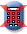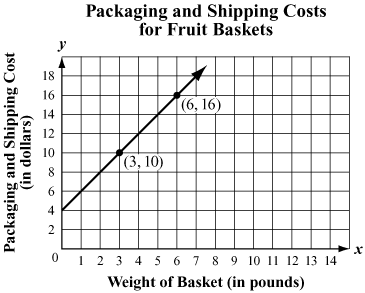Massachusetts Comprehensive Assessment SystemReporting Category: Algebra and Functions
Standard: 10.P.2 - Demonstrate an understanding of the relationship between various representations of a line. Determine a line's slope and x- and y-intercepts from its graph or from a linear equation that represents the line. Find a linear equation describing a line from a graph or a geometric description of the line, e.g., by using the "point-slope" or "slope y-intercept" formulas. Explain the significance of a positive, negative, zero, or undefined slope. (AI.P.5)
Standard: CCSS.Math.Content.HSF-IF.B.4 - For a function that models a relationship between two quantities, interpret key features of graphs and tables in terms of the quantities, and sketch graphs showing key features given a verbal description of the relationship. Key features include: intercepts; intervals where the function is increasing, decreasing, positive, or negative; relative maximums and minimums; symmetries; end behavior; and periodicity.*

A company packages fruit baskets of different weights and ships them to customers. The company charges a flat fee for packaging the baskets. The total packaging and shipping cost in dollars, y, of a fruit basket weighing x pounds is represented by the line on the graph below.1. What is the y-intercept of the line on the graph?
2. What does the y-intercept of the line represent in this situation?
3. What is the slope of the line on the graph? Show or explain how you got your answer.
4. What does the slope of the line represent in this situation?
5. Write an equation that represents the line on the graph.
6. Use the equation you wrote in part (e) to determine the weight, in pounds, of the heaviest fruit basket that could be packaged and shipped for \$50. Show or explain how you got your answer.

## Scoring Guide and Sample Student Work

Select a score point in the table below to view the sample student response.

ScoreDescription
4 The student response demonstrates an exemplary understanding of the Functions concepts involved in interpreting, for a function that models a relationship between two quantities, key features of graphs in terms of the quantities. The student interprets the meaning of the slope and the y-intercept and defines their relationship in a real-world situation.
4
3 The student response demonstrates a good understanding of the Functions concepts involved in interpreting, for a function that models a relationship between two quantities, key features of graphs in terms of the quantities. Although there is significant evidence that the student was able to recognize and apply the concepts involved, some aspect of the response is flawed. As a result the response merits 3 points.
2 The student response demonstrates a fair understanding of the Functions concepts involved in interpreting, for a function that models a relationship between two quantities, key features of graphs in terms of the quantities. While some aspects of the task are completed correctly, others are not. The mixed evidence provided by the student merits 2 points.
1 The student response demonstrates a minimal understanding of the Functions concepts involved in interpreting, for a function that models a relationship between two quantities, key features of graphs in terms of the quantities.
0 The student response contains insufficient evidence of an understanding of the Functions concepts involved in interpreting, for a function that models a relationship between two quantities, key features of graphs in terms of the quantities to merit any points.
Note: There are 2 sample student responses for Score Point 4.

Question 17: Number and Quantity
Question 20: Algebra and Functions
Question 21: Algebra and Functions
Question 36: Geometry
Question 41: Statistics and Probability
Question 42: Geometry

Last Updated: April 26, 2022